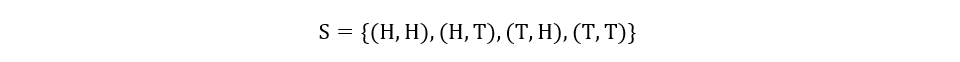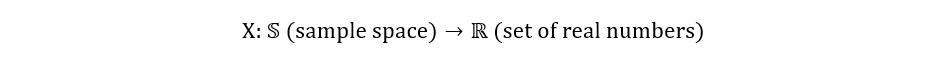Naman Agrawal — May 14, 2021

This article was published as a part of the Data Science Blogathon.

## What are Random Variables?

A random variable (also known as a stochastic variable) is a real-valued function, whose domain is the entire sample space of an experiment. Think of the domain as the set of all possible values that can go into a function. A function takes the domain/input, processes it, and renders an output/range. Similarly, a random variable takes its domain (sample space of an experiment), processes it, and assigns every event/outcome a real value. This set of real values obtained from the random variable is called its range.

In statistical notations, a random variable is generally represented by a capital letter, and its realizations/observed values are represented by small letters.

Consider the experiment of tossing two coins. We can define X to be a random variable that measures the number of heads observed in the experiment. For the experiment, the sample space is shown below:There are 4 possible outcomes for the experiment, and this is the domain of X. The random variable X takes these 4 outcomes/events and processes them to give different real values. For each outcome, the associated value is shown as:Thus, we can represent X as follows:## Types of Random Variables

There are three types of random variables- discrete random variables, continuous random variables, and mixed random variables.

1) Discrete Random Variables: Discrete random variables are random variables, whose range is a countable set. A countable set can be either a finite set or a countably infinite set. For instance, in the above example, X is a discrete variable as its range is a finite set ({0, 1, 2}).

2) Continuous Random Variables: Continuous random variables, on the contrary, have a range in the forms of some interval, bounded or unbounded, of the real line. E.g., Let Y be a random variable that is equal to the height of different people in a given population set. Since the people can have different measures of height (not limited to just natural numbers or any countable set), Y is a continuous variable (in fact, the distribution of Y follows a normal/gaussian distribution on most occasions).

3) Mixed Random Variables: Lastly, mixed random variables are ones that are a mixture of both continuous and discrete variables. These variables are more complicated than the other two. Hence, they are explained at the end of this article.

## Probability Distribution of Random Variables

When we describe the values in the range of a random variable in terms of the probability of their occurrence, we are essentially talking about the probability distribution of the random variable. In other words, the probability distribution of a random variable can be determined by calculating the probability of occurrence of every value in the range of the random variable. A probability distribution is described for discrete and continuous random variables in subtly different ways.

### The Discrete Case

For discrete variables, the term ‘Probability mass function (PMF)’ is used to describe their distributions. Using the example of coin tosses, as discussed above, we calculate the probability of X taking the values 0, 1 and 2 as follows:

We use the notation PX(x) to refer to
the PMF of the random variable X. The distribution is shown as follows:

The table can also be graphically demonstrated:

In general, if a random variable X has a countable range given by:

Then, we define probability mass function as:

This also leads us to the general description of the distribution in tabular format:

Properties of probability mass function:

1) PMF can never be more than 1 or negative i.e.,

2) PMF must sum to one over the entire range set of a random variable.

3) For A, a subset of Rx,

### The Continuous Case

For continuous variables, the term ‘Probability density function (PDF)’ is used to describe their distributions. We’ll consider the example of the distribution of heights. Suppose, we survey a group of 1000 people and measure the height of each person very precisely. The distribution of the heights can be shown by a density histogram as follows:

We have grouped the different heights in certain intervals. But let’s see what happens when we try to reduce the size of the histogram bins. In other words, we make the grouping intervals smaller and smaller.

Going further, we further reduce the bin size to such an extent that every observation tends to have its own bin. We are essentially constructing these extremely tiny rectangles, that we connect together by a smooth curve, giving us the following distribution:

And that’s it! We have got the probability distribution of heights for our sample population set. But how’s probability related to all of this? Observe the y axis. It shows density, which indicates the proportion of the population having a particular range of height. The probability that a randomly chosen person from the population having a height within the given interval corresponds to this proportion. That sounds more probabilistic!

We use the notation fX(x) to refer to the PDF of random variable X. Both PMF and PDF are analogous. We just replace summation with integration to account for their continuous behaviour.

Properties of probability density function:

1) PDF can never negative i.e.,

2) PDF must integrate to one over the entire range of a random variable.

3) For A, a subset of Rx,

More specifically, if A = [a, b], then,

Graphically, the probability that a continuous random variable X takes a value within a given interval is the area below the PDF for X, enclosed between the given interval. For instance, in the above example, if we wish to determine the probability that a randomly selected person from the population has a height between 65 cm and 75 cm, we calculate the purple area (using definite integration):

Note: Unlike PMF, PDF can take a value greater than 1. This is because of a difference in their interpretation. In the case of PMF, the value of the function for a particular x has the same interpretation as probability, making its value restricted to the [0, 1] interval. However, in PDF, the value does not translate to probability. In fact, P(X = x) = 0, if X is a continuous variable (it’s like calculating area under the PDF curve, just below a point).

## Cumulative Distribution of Random Variables

Sometimes, it’s easier to have the distribution of a random variable expressed in an alternative way. Cumulative distribution functions (CDF) are one such way. Cumulate means to gather or sum up. CDFs do the same. A useful property of CDFs is that they are defined in the same way for both discrete and continuous variables. A CDF shows the probability that a random variable X takes a value lesser than or equal to x. Mathematically, a CDF is defined as follows:

Let’s consider both the discrete and the continuous case.

### The Discrete Case

The CDF of discrete random variables resembles a staircase, a graph with many jumps. We’ll again use the coin toss example. The following PMF was obtained:

We’ll now calculate the CDF of X for different values of x:

Hence, we can define FX(x) as follows:

The CDF can also be shown graphically as follows:

For discrete variables, we can define the following relation between PMF and CDF:

### The Continuous Case

The CDF of continuous random variables is noisier than that of discrete variables. Just as previously done, we sum up (rather say, integrate) the PDF to get the CDF. For the example of the height, the following CDF has been made:

For continuous variables, we can define the following relation between PDF and CDF:

### Properties of CDF

1) CDF is always a non-decreasing function.

2) CDF always lies between 0 and 1.

3) For all a < b,

## Expectation & Variance of Random Variables

Many times, it’s handy to use just a few numbers to express the distribution of a random variable. There are many such numbers- most common of which are expectation and variance.

### The Expectation of Random Variables

The expectation (also known as mean) of a random variable X is its weighted average. For discrete random variables, the expectation is calculated using the following equation:

For continuous variables, once again, we replace summation with integration to arrive at the following equation:

Basic properties of expectation of random variables:

1) The expectation of a constant is the constant itself.

2) The expectation of the sum of two random variables is equal to the sum of their expectations.

3) If Y = aX + b, then the expectation of Y is calculated as:

### The Variance of Random Variables

The variance of a random variable X is the expected value of the square of the deviation of different values of X from the expectation of X. It shows the spread of the distribution of a random variable is. It is generally represented as:

However, a more useful expression for variance is obtained after simplifying the above equation:

Where,

Basic properties of variance of random variables:

1) The variance of a constant is zero.

2) For two random variables- X & Y, the variance of their sum is expressed as follows:

Cov(X, Y) is called the covariance of X & Y. Covariance describes the relationship between two variables. It can be defined by the following equation:

3) If Y = aX + b, then the variance of Y is defined as:

## Mixed Random Variables

Mixed random variables have a discrete part (where the range of the variable is a countable set), and a continuous part (where the range of the variable takes the form of an interval of the real line). A mixed random variable Z can be shown as follows:

The CDF of the mixed random variable Z can be found out by calculating the weighted average of its components:

The expectation of Z can also be calculated by using the above methodology:

## The Bottom Line

This concludes our discussion on ‘Understanding Random Variables & their Distributions’. While this might have been an intensive read, it’s imperative to acknowledge that the study of random variables isn’t restricted to just the above-explained concepts. Various other topics such as the independence of random variables, their joint distributions, and transformations are also relevant for further deepening of our understanding of them.

The media shown in this article are not owned by Analytics Vidhya and is used at the Author’s discretion.